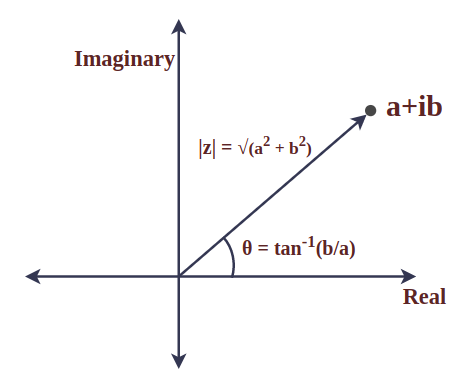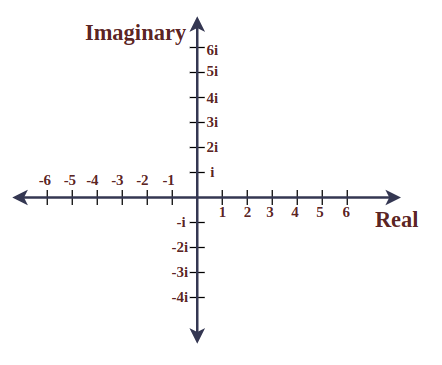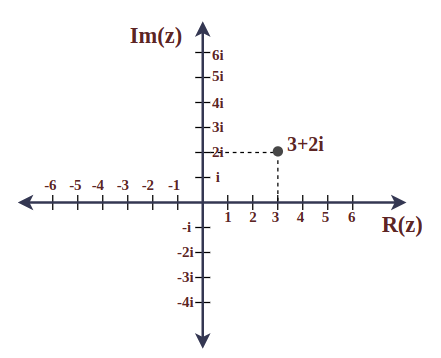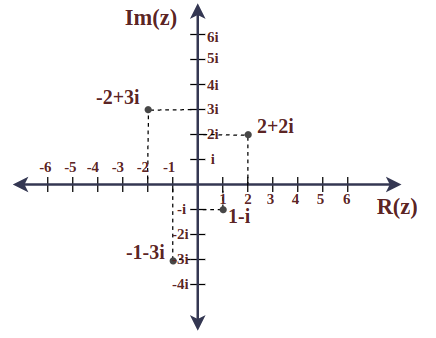GeeksforGeeks App
Open AppBrowser
Continue

## Related Articles

Complex Numbers are the natural continuation of real numbers. In the story of numbers complex numbers took a long time to be well-reconsigned. A lot of mathematicians, thinkers, and philosophers hesitated for a long time to believe in the existence of complex or imaginary numbers. But slowly and steadily as complex numbers seem beneficial in many advanced mathematical problems to many mathematical minds, these number got their place in the numbers and completed the numbers. In the modern age complex number are used in many fields such as digital signal processing, cryptography, and many computer-related fields. In this article, we will learn about imaginary numbers, complex numbers, and its type, various operations on complex numbers, properties of complex numbers, application of complex numbers, etc.

## Complex Numbers Definition

Complex Numbers are the numbers of the form (a + i b) where a & b are the real numbers and i is an imaginary unit called iota that represents √-1. For example, 2 + 3i, is a complex number in which 2 is a real number and 3i is an imaginary number.### Modulus of Complex Number

Modulus of the Complex Number is the absolute value and represents the distance between the origin and the given point. It is also known as the magnitude of the complex number. Let’s consider a complex number z = a + ib then the modulus of z is defined as:

|z| = √(a2 + b2)

Where,

• a is the real part of the complex number z, and
• b is the imaginary part of the complex number z.

### Argument of Complex Number

The angle between the radius vector of a complex number and the positive x-axis is called the argument of a complex number. For a complex number z = a + ib, it is mathematically given by:

θ = tan-1(b/a)

Where,

• a is the real part of the complex number z, and
• b is the imaginary part of the complex number z.

### Power of i(iota)

The “i(iota)” is defined as the square root of -1. Thus, any power of “i” can be expressed as a repeated multiplication of “i” by itself i.e.,

• i = √(-1)
• i2 = -1
• i3 = – i
• i4 =  1
• i5 =  i
• i6 = – 1
• and so on..

## Need for Complex Numbers

In ancient times, people only had knowledge of natural numbers as these numbers are most intuitive in nature as the human brain already has an understanding of them using visuals of things such as sheep and food. Thus, we only have the set of natural numbers (N) but in natural numbers, there is no solution to the equation x + a = b (a>b) and a, b ∈ N. Thus, an extension of natural numbers came into existence i.e., Integers(I).

Now, again in this set of numbers, there is no solution to the equation, ax = b (a ≠ 0) and a, b ∈ I, where a and b both are integers. Thus, a set of integers (I) is extended to a set of rational numbers (Q).

Again, in this set of rational numbers, there is no solution to the equation x2 = a (a > 0) and a ∈ Q. Thus, Q is extended to include numbers such that, x2 = a(for a > 0) i.e., irrational numbers. This set is named Real Numbers and is represented by R.

Now, it was thought for a long time that we don’t have to extend this set of real numbers to form another bigger set as this collection of numbers seems complete. But again a new problem aroused in this set of numbers i.e., there is no real number such that x2 = a (a < 0) and a ∈ R. Thus, the set of real numbers is extended further to include all such valued and named this set Complex Numbers and is represented by C.

## Classification of Complex Numbers

As we know the standard form of a complex number is z = (a + i b) where a, b ∈ R, and i is iota (an imaginary unit). So depending on the values of a (called real part) and b (called imaginary part), the complex numbers are classified into four types:

• Zero Complex Number
• Purely Real Numbers
• Purely Imaginary Numbers
• Imaginary Numbers

Let’s learn about these types in detail.

### Zero Complex Number

For any complex number z = a + ib if a = 0 & b = 0, then the complex number is called zero complex number. For example, the only example of this is 0.

### Purely Real Numbers

For any complex number z = a + ib if a ≠ 0 & b = 0, then the complex number is called a purely real number i.e., a number with no imaginary part. All the real numbers are examples of this such that 2, 3, 5, 7, etc.

### Purely Imaginary Numbers

For any complex number z = a + ib if a = 0 & b ≠ 0, then a complex number is called a purely imaginary number i.e., a number with no real part. All numbers with no real parts are examples of this type of number i.e.,  -7i, -5i, -i, i, 5i, 7i, etc.

### Imaginary Numbers

For any complex number z = a + ib if a ≠ 0 & b ≠ 0, then a complex number is called an imaginary number. For example,  (-1 – i), (1 + i), (1 – i), (2 + 3i), etc.

## Different Forms of Complex Numbers

There are various forms of complex numbers that are,

• Rectangular Form
• Polar Form
• Exponential Form

Now let’s learn about them in detail.

### Rectangular Form

Rectangular Form is also called Standard Form and it is represented by (a + ib), where a and b are the real numbers.

For example: (5 + 5i), (-7i), (-3 – 4i), etc.

### Polar Form

Polar Form is the representation of a complex number where  polar coordinates [where coordinates are represented as (r, θ), where r is the distance from the origin and θ is the angle between the line joining the point and origin and the positive x-axis) are used to represent a complex number. Any complex number is represented as r [cos θ + i sin θ].

For examples: [cos π/2 + i sin π/2], 5[cos π/6 + i sin π/6], etc.

### Exponential Form

Exponential Form is the different representation of polar form using Euler’s Formula and in this form complex number is represented by re, where r is the distance of a point from the origin and θ is the angle between the positive x-axis and radius vector.

For examples: ei(0), ei(π/2), 5.ei(π/6), etc.

Note: All three forms of the complex numbers discussed above are interconvertible i.e., these can be converted from one form to another very easily.

## Operations on Complex Numbers

The following operations can be performed on Complex Numbers:

• Subtraction
• Multiplication
• Division
• Conjugation

We can add two complex numbers, by simply adding their real and imaginary parts separately.

For example, (3 + 2i) + (1 + 4i) = 4 + 6i.

### Subtraction of Complex Numbers

We can subtract two complex numbers, by simply subtracting their real and their imaginary parts separately.

For example, (3 + 2i) – (1 + 4i) = 2 – 2i.

### Multiplication of Complex Numbers

We can multiply two complex numbers using the distributive property and the fact that i2 = -1.

For example, (3 + 2i)(1 + 4i) = 3 + 12i + 2i + 8i2 = 3 + 14i – 8 = -5 + 14i.

### Division of Complex Numbers

We can divide one complex number by another, by simply multiplying both the numerator and the denominator by the complex conjugate of the denominator and further simplifying the expression.

For example, (3 + 2i)/(1 + 4i) = (3 + 2i)(1 – 4i)/(1 + 4i)(1 – 4i) = (11 – 10i)/17.

### Conjugation of Complex Numbers

We can easily find the conjugate of a complex number, by simply changing the sign of its imaginary part.

For example, the conjugate of 3 + 2i is 3 – 2i.

Note: The conjugate of a complex number is often denoted with a bar above the number, such as z̄.

## Identities for Complex Numbers

For any two complex numbers zand z2 the following algebraic  identities can be given:

• (z+ z2)2 = (z1)+ (z2)2 + 2 z1 × z2
• (z1 – z2)2 = (z1)+ (z2)2 – 2 z1 × z2
• (z1)2 – (z2)2 =  (z+ z2)(z1 – z2)
• (z+ z2)3 = (z1)+ 3(z1)2 z +3(z2)2 z+ (z2)3
• (z– z2)3 = (z1)– 3(z1)2 z +3(z2)2 z– (z2)3

## Formulas Related to Complex Numbers

There are some formulas related to complex numbers, some of which are as follows:

### Euler’s Formula

Euler’s Formula shows the relation between the imaginary power of exponent and trigonometric ratio sin and cos and is given by:

eix = cos x + i sin x

### De Moivre’s Formula

De Moivre’s Formula expresses the nth power of a complex number in polar form and is given by:

(cos x + i sin x)n = cos (nx) + i sin (nx)

## Complex Plane

The plane on which the complex numbers are uniquely represented is called the Complex plane or Argand plane or Gaussian plane.

The Complex plane has two axes:

• X-axis or Real Axis
• Y-axis or Imaginary Axis

X-axis or Real Axis

• All the purely real complex numbers are uniquely represented by a point on it.
• Real part Re(z) of all complex numbers are plotted with respect to it.
• That’s why X-axis is also called Real axis.

Y-axis or Imaginary Axis

• All the purely imaginary complex numbers are uniquely represented by a point on it.
• Imaginary part Im(z) of all complex numbers are plotted with respect to it.
• That’s why Y-axis is also called Imaginary axis.## Geometrical Representation of Complex Numbers

As we know that every complex number (z = a + i b) is represented by a unique point p(a, b) on the complex plane and every point on the complex plane represents a unique complex number.

To represent any complex number z = (a + i b) on the complex plane follow these conventions:

• Real part of z (Re(z) = a) becomes the X-coordinate of the point p
• Imaginary part of z (Im(z) = b) becomes the Y-coordinate of the point p

And finally z (a + i b) ⇒ p (a, b) which is a point on the complex plane.

## Properties of Complex Numbers

There are various properties of complex numbers, some of which are as follows:

• For any complex number z = a + ib, if z = 0 then a = 0 as well as b = 0.
• For 4 real numbers a, b, c, and d such that z1 = a + ib and  z2 = c + id. If z1 = z2 then a = c, and b=d.
• The addition of a complex number with its conjugate results in a purely real number i.e., z + z̄ = Real Number.

Let z = a + ib,

z + z̄ = a + ib  + a – ib

z + z̄ = 2a (which is purely real)

• The product of a complex number with its conjugate results is a purely real number as well i.e.,  z × z̄ = Real Number

Let z = a + ib, then

z × z̄ = (a + ib) × (a – ib)

⇒ z × z̄= a2 – i2b2

⇒ z × z̄ = a2 + b2 (which is purely real)

• Complex Numbers are commutative under the operation of addition and multiplication. Let’s consider two complex numbers  z1 and  z2, and then

z1+z2  = z2+z1

z1× z2  = z2× z1

• Complex Numbers are associative with the operation of addition and multiplication. Let’s consider three complex numbers  z1, z2, and z3  then

(z1+z2) +z3 = z1 + (z2+z3)

(z1×z2)×z3 = z1×(z2×z3)

• Complex Numbers hold the distributive property of multiplication over addition as well. Let’s consider three complex numbers  z1, z2, and z3  then

z1×(z2+z3) = z1×z2 + z1×z3

## Solved Examples on Complex Numbers

Example 1: Plot these complex numbers z = 3 + 2i on the Complex plane.

Solution:

Given:

z = 3 + 2 i

So, the point is z(3, 2). Now we plot this point on the below graph, here in this graph x-axis represents the real part and y-axis represents the imaginary part.Example 2: Plot these complex numbers z1 = (2 + 2 i), z2 = (-2 + 3 i), z3 = (-1 – 3 i), z4 = (1 – i) on the Complex plane.

Solution:

Given:

z1 = (2 + 2 i)

z2 = (-2 + 3 i)

z3 = (-1 – 3 i)

z4 = (1 – i)

So, the points are z1 (2, 2), z2(-2, 3), z3(-1, -3), and z4(1, -1). Now we plot these points on the below graph, here in this graph x-axis represents the real part and y-axis represents the imaginary part.## FAQs on Complex Numbers

### Q1: Define complex numbers.

Numbers of the form a+ib is called complex number, where a and b are the real number and i is the imaginary unit which represents square root of -1.

### Q2: What is the difference between a real number and a complex number?

The key difference between real and complex numbers is that we only need one number to represent any real number but need two real numbers to represent any complex number.

### Q3: What is the real part and imaginary part of a complex number?

In a complex number a + ib, a is the real part of the complex number, and b is called the imaginary part of the complex number.

### Q4: What is the complex conjugate of a complex number?

For a complex number a + ib, a – ib is called its complex conjugate. Complex conjugates can be found by simply changing the sign of the imaginary part.

### Q5: What is the modulus of a complex number?

The distance between the origin and the point represented by a complex number in the argand plane is called the modulus of that complete number and for z = a + ib, it is mathematically given by:

|z| = √(a2 + b2)

Where,

• a is the real part of the complex number z, and
• b is the imaginary part of the complex number z.

### Q6: What is the argument of a complex number?

The angle between the radius vector of a complex number and the positive x-axis is called the argument of a complex number and for z = a + ib, it is mathematically given by:

θ = tan-1(b/a)

Where,

• a is the real part of the complex number z, and
• b is the imaginary part of the complex number z.

### Q7: What is the polar form of a complex number?

For any complex number, z = a + ib, the polar form of this is given by:

r [cos θ + i sin θ]

Where,

r is the modulus of the complex number, and

θ is the argument of the complex number.